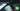# Hackerrank | Solution of Mini-Max Sum in Golang

September 2nd, 2018
|

In this post we will solve question mini-max sum from hackerrank. Here we will learn a bit about make in golang along with a new golang package called sort. Let's get started.

# Problem Statement

The question can be found at this link. The problem statement basically states that we are given an array of five integers, and we need to print maximum possible sum of any four numbers and minimum possible sum of any four numbers. Couple of things to note here is

• We need to take an array as input.
• Array length is fixed to be five
• we are solving this in golang, and we will be using `make` to create our array. So if you are unfamiliar with this please refer to my previous posts, however I'll try to explain it again in this article.

# Challenges

• Choose our preferred language as golang on hackerrank. The moment we do that, we get some 50-60 lines of code which are very unfamiliar to someone who is new to language.
• Some Golang constraints that we will see in a couple of minutes.

# Solution

This is the template you get on hackerrank for this problem statement.

``````    package main

import (
"bufio"
"fmt"
"io"
"os"
"strconv"
"strings"
)

// Complete the miniMaxSum function below.
func miniMaxSum(arr []int32) {

}

func main() {

var arr []int32

for i := 0; i < 5; i++ {
arrItemTemp, err := strconv.ParseInt(arrTemp[i], 10, 64)
checkError(err)
arrItem := int32(arrItemTemp)
arr = append(arr, arrItem)
}

miniMaxSum(arr)
}

if err == io.EOF {
return ""
}

return strings.TrimRight(string(str), "\r\n")
}

func checkError(err error) {
if err != nil {
panic(err)
}
}``````
• First go ahead and delete everything in the pre-existing template.
• Let's write down our main wrapper function.
``````package main
import "fmt"

func main(){

}``````

First thing we need is an array to store our elements. We won't be taking inputs in a temporary variable, since we need to do some sorting operation on the array later on. If you remember from the earlier posts we used `var a int` to declare an array, and we have also seen why this approach only works for constants. This time we have a constant length i.e. 5 for the array, still we are going to use `make` to allocate the memory for array and will not use `[]int` declaration. Please bear with me for sometime, and I'll explain the reason behind the decision. So let's go ahead declare our array.

``````    ...
a := make([]int, 5)

}``````

Here we are creating an array that will hold integer type of data and will be of length 5. Next we need to take inputs for this array. Let's go ahead and do that.

``````    ...
fmt.Scan(&a,&a,&a,&a,&a)``````

Now that we have our array ready let's think about the operations, we need to do. If we think through it, max sum of 4 elements and min sum of four elements can be easily calculated once we sort the array in increasing order. The sum of first four elements will be the min sum, and sum of last four elements will be max sum. So now the task at hand is to sort the array we have. To sort an array we will make use of `sort` package from golang, and to be precise `Ints` function from sort package. So let's change our import statement to add sort package

``````    ...
import (
"fmt"
"sort"
)
...``````

Now let's sort the array after the input statement.

``````    ...
sort.Ints(a)``````

Now that we have our array sorted, we need to calculate minimum and maximum sum and print it. Let's do that and wrap up this post.

``````    ...
min := a+a+a+a
max := a+a+a+a
fmt.Println(min,max)``````

Pretty straight forward, calculating min sum, max sum and printing it. Now our complete code should look something like

``````    package main

import (
"fmt"
"sort"
)

func main(){

a := make([]int, 5)

fmt.Scan(&a,&a,&a,&a,&a)
sort.Ints(a)
min := a+a+a+a
max := a+a+a+a
fmt.Println(min,max)
}``````

Now that we have a working solution, let's discuss why we didn't go with our `[]int` declaration and instead went ahead with `make`. So in the first case we get an array while in second case we get a slice which refers to an array. What are slices? Slices are just like arrays, but their length is not fixed. They can grow and shrink. So why did we need slices? The reason is very simple, the `Ints` function from `sort` package takes a slice as an argument instead of an array, thus we went ahead and used a slice. Can we still solve this question using arrays? Yes, sort the array yourself. The length is very small, even if you use selection sort, no worries. Is there any other way to declare and work with slices? Yes we will look into some very soon. This is it for this one, complete source code for this post can be found on my Github Repo. Will see you in the next one.